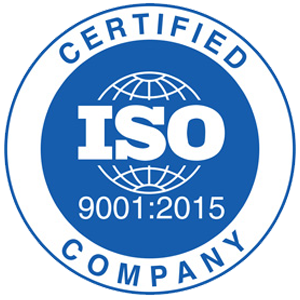Phone Number: 800-867-3526
1-800-TORELCO

Email: info@torelco.com

# Understanding and Calculating Inductance of Toroids

All electrical engineers and technicians encounter inductors regularly as a result of their work. Learning about inductance and related parameters ensures that systems run optimally. Even if you do not specialize in manufacturing inductors, it is important for those using them to have a basic understanding of their functions and operational principles.It is common for the varying electric currents to flow through a coil. For example, an AC flow creates a change in the magnetic field. The resulting change in magnetic fields induces a secondary current. The secondary current is in opposition to the source current. Simply put, inductance (self-inductance) is defined as the ability of an electric circuit to opposes changes to the current that is flowing through the system.

The equation to determine inductance is e = –L(di/dt). Inductance is represented as L in the equation, and it is the amount that rate of change in currents that are multiplied by to obtain the EMF (electromagnetic force). L is the constant, and it refers to the coefficient of self-induction, which is measures in Henrys (H). It is considered negative in the equation to indicate that inductance voltage has an opposite polarity from the source of voltage.

The total amount of inductance within a coil gets determined by the number of fluxes that links the coil. The coil size determines flux. Custom coil winding also affects flux. Another important factor to consider is the presence, or lack thereof, of a core’s magnetic material.